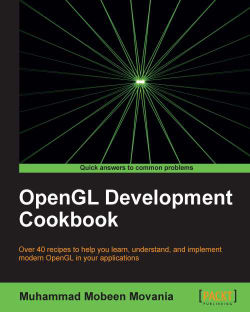# OpenGL Development Cookbook5 (5 reviews total)
• \$30.99 eBook
• \$12.99 eBook + Subscription
What do you get with a Packt Subscription?

• Constantly updated with 100+ new titles each month
• Breadth and depth in over 1,000+ technologies

OpenGL is the leading cross-language, multi-platform API used by masses of modern games and applications in a vast array of different sectors. Developing graphics with OpenGL lets you harness the increasing power of GPUs and really take your visuals to the next level.

OpenGL Development Cookbook is your guide to graphical programming techniques to implement 3D mesh formats and skeletal animation to learn and understand OpenGL.

OpenGL Development Cookbook introduces you to the modern OpenGL. Beginning with vertex-based deformations, common mesh formats, and skeletal animation with GPU skinning, and going on to demonstrate different shader stages in the graphics pipeline. OpenGL Development Cookbook focuses on providing you with practical examples on complex topics, such as variance shadow mapping, GPU-based paths, and ray tracing. By the end you will be familiar with the latest advanced GPU-based volume rendering techniques.

Publication date:
June 2013
Publisher
Packt
Pages
326
ISBN
9781849695046

## Chapter 1. Introduction to Modern OpenGL

In this chapter, we will cover:

• Setting up the OpenGL v3.3 core profile on Visual Studio 2010 using the GLEW and freeglut libraries

• Designing a GLSL shader class

• Rendering a simple colored triangle using shaders

• Doing a ripple mesh deformer using the vertex shader

• Dynamically subdividing a plane using the geometry shader

• Dynamically subdividing a plane using the geometry shader with instanced rendering

• Drawing a 2D image in a window using the fragment shader and SOIL image loading library

## Introduction

The OpenGL API has seen various changes since its creation in 1992. With every new version, new features were added and additional functionality was exposed on supporting hardware through extensions. Until OpenGL v2.0 (which was introduced in 2004), the functionality in the graphics pipeline was fixed, that is, there were fixed set of operations hardwired in the graphics hardware and it was impossible to modify the graphics pipeline. With OpenGL v2.0, the shader objects were introduced for the first time. That enabled programmers to modify the graphics pipeline through special programs called shaders, which were written in a special language called OpenGL shading language (GLSL).

After OpenGL v2.0, the next major version was v3.0. This version introduced two profiles for working with OpenGL; the core profile and the compatibility profile. The core profile basically contains all of the non-deprecated functionality whereas the compatibility profile retains deprecated functionality for backwards compatibility. As of 2012, the latest version of OpenGL available is OpenGL v4.3. Beyond OpenGL v3.0, the changes introduced in the application code are not as drastic as compared to those required for moving from OpenGL v2.0 to OpenGL v3.0 and above.

In this chapter, we will introduce the three shader stages accessible in the OpenGL v3.3 core profile, that is, vertex, geometry, and fragment shaders. Note that OpenGL v4.0 introduced two additional shader stages that is tessellation control and tessellation evaluation shaders between the vertex and geometry shader.

## Setting up the OpenGL v3.3 core profile on Visual Studio 2010 using the GLEW and freeglut libraries

We will start with a very basic example in which we will set up the modern OpenGL v3.3 core profile. This example will simply create a blank window and clear the window with red color.

OpenGL or any other graphics API for that matter requires a window to display graphics in. This is carried out through platform specific codes. Previously, the GLUT library was invented to provide windowing functionality in a platform independent manner. However, this library was not maintained with each new OpenGL release. Fortunately, another independent project, freeglut, followed in the GLUT footsteps by providing similar (and in some cases better) windowing support in a platform independent way. In addition, it also helps with the creation of the OpenGL core/compatibility profile contexts. The latest version of freeglut may be downloaded from http://freeglut.sourceforge.net. The version used in the source code accompanying this book is v2.8.0. After downloading the freeglut library, you will have to compile it to generate the libs/dlls.

The extension mechanism provided by OpenGL still exists. To aid with getting the appropriate function pointers, the GLEW library is used. The latest version can be downloaded from http://glew.sourceforge.net. The version of GLEW used in the source code accompanying this book is v1.9.0. If the source release is downloaded, you will have to build GLEW first to generate the libs and dlls on your platform. You may also download the pre-built binaries.

Prior to OpenGL v3.0, the OpenGL API provided support for matrices by providing specific matrix stacks such as the modelview, projection, and texture matrix stacks. In addition, transformation functions such as translate, rotate, and scale, as well as projection functions were also provided. Moreover, immediate mode rendering was supported, allowing application programmers to directly push the vertex information to the hardware.

In OpenGL v3.0 and above, all of these functionalities are removed from the core profile, whereas for backward compatibility they are retained in the compatibility profile. If we use the core profile (which is the recommended approach), it is our responsibility to implement all of these functionalities including all matrix handling and transformations. Fortunately, a library called `glm` exists that provides math related classes such as vectors and matrices. It also provides additional convenience functions and classes. For all of the demos in this book, we will use the `glm` library. Since this is a headers only library, there are no linker libraries for `glm`. The latest version of `glm` can be downloaded from http://glm.g-truc.net. The version used for the source code in this book is v0.9.4.0.

There are several image formats available. It is not a trivial task to write an image loader for such a large number of image formats. Fortunately, there are several image loading libraries that make image loading a trivial task. In addition, they provide support for both loading as well as saving of images into various formats. One such library is the `SOIL` image loading library. The latest version of `SOIL` can be downloaded from http://www.lonesock.net/soil.html.

Once we have downloaded the `SOIL` library, we extract the file to a location on the hard disk. Next, we set up the include and library paths in the Visual Studio environment. The include path on my development machine is `D:\Libraries\soil\Simple OpenGL Image Library\src` whereas, the library path is set to `D:\Libraries\soil\Simple OpenGL Image Library\lib\VC10_Debug`. Of course, the path for your system will be different than mine but these are the folders that the directories should point to.

These steps will help us to set up our development environment. For all of the recipes in this book, Visual Studio 2010 Professional version is used. Readers may also use the free express edition or any other version of Visual Studio (for example, Ultimate/Enterprise). Since there are a myriad of development environments, to make it easier for users on other platforms, we have provided premake script files as well.

The code for this recipe is in the `Chapter1/GettingStarted` directory.

### Tip

You can download the example code files for all Packt books you have purchased from your account at http://www.packtpub.com. If you purchased this book elsewhere, you can visit http://www.packtpub.com/support and register to have the files e-mailed directly to you.

### How to do it...

Let us setup the development environment using the following steps: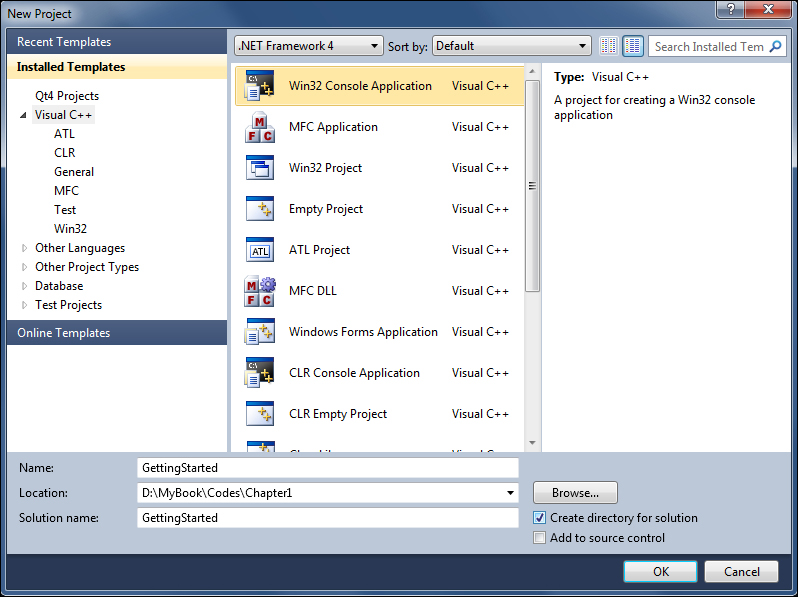2. We first create a new Win32 Console Application project as shown in the preceding screenshot. We set up an empty Win32 project as shown in the following screenshot: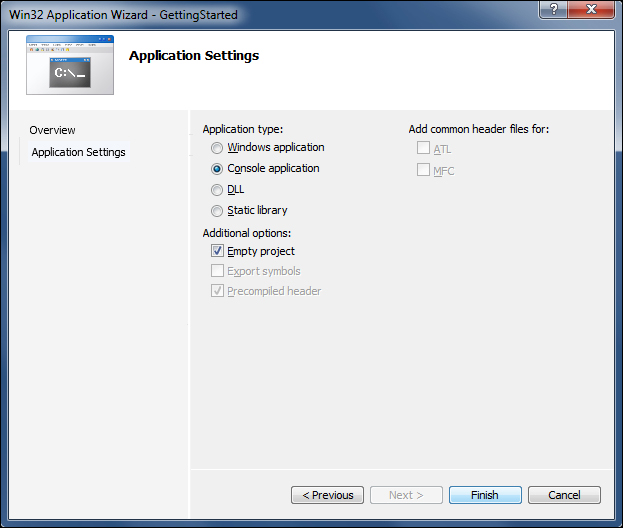3. Next, we set up the include and library paths for the project by going into the Project menu and selecting project Properties. This opens a new dialog box. In the left pane, click on the Configuration Properties option and then on VC++ Directories.

4. In the right pane, in the Include Directories field, add the GLEW and freeglut subfolder paths.

5. Similarly, in the Library Directories, add the path to the lib subfolder of GLEW and freeglut libraries as shown in the following screenshot: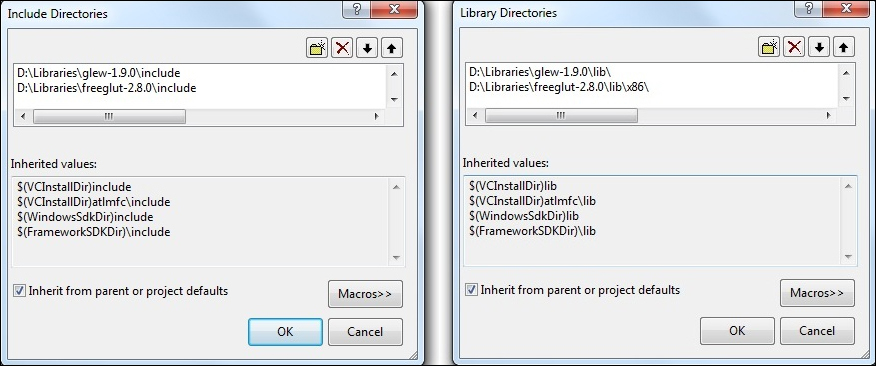6. Next, we add a new `.cpp` file to the project and name it `main.cpp`. This is the main source file of our project. You may also browse through `Chapter1/ GettingStarted/GettingStarted/main.cpp` which does all this setup already.

7. Let us skim through the `Chapter1/ GettingStarted/GettingStarted/main.cpp` file piece by piece.

```#include <GL/glew.h>
#include <GL/freeglut.h>
#include <iostream>```

These lines are the include files that we will add to all of our projects. The first is the GLEW header, the second is the freeglut header, and the final include is the standard input/output header.

8. In Visual Studio, we can add the required linker libraries in two ways. The first way is through the Visual Studio environment (by going to the Properties menu item in the Project menu). This opens the project's property pages. In the configuration properties tree, we collapse the Linker subtree and click on the Input item. The first field in the right pane is `Additional Dependencies`. We can add the linker library in this field as shown in the following screenshot: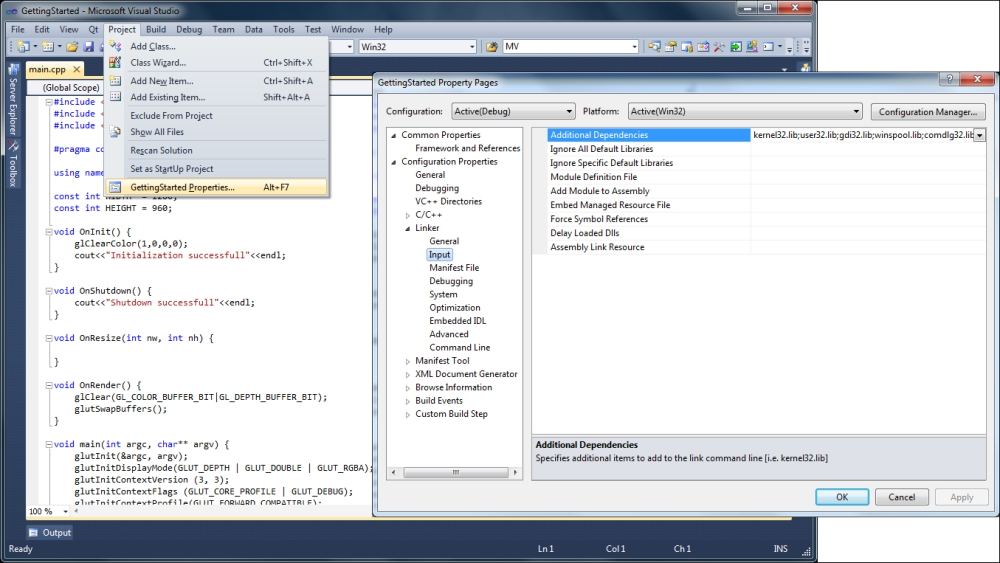9. The second way is to add the `glew32.lib` file to the linker settings programmatically. This can be achieved by adding the following `pragma`:

`#pragma comment(lib, "glew32.lib")`
10. The next line is the using directive to enable access to the functions in the std namespace. This is not mandatory but we include this here so that we do not have to prefix `std::` to any standard library function from the iostream header file.

`using namespace std;`
11. The next lines define the width and height constants which will be the screen resolution for the window. After these declarations, there are five function definitions . The `OnInit()` function is used for initializing any OpenGL state or object, `OnShutdown()` is used to delete an OpenGL object, `OnResize()` is used to handle the resize event, `OnRender()` helps to handle the paint event, and `main()` is the entry point of the application. We start with the definition of the `main()` function.

```const int WIDTH  = 1280;
const int HEIGHT = 960;

int main(int argc, char** argv) {
glutInit(&argc, argv);
glutInitDisplayMode(GLUT_DEPTH | GLUT_DOUBLE | GLUT_RGBA);
glutInitContextVersion (3, 3);
glutInitContextFlags (GLUT_CORE_PROFILE | GLUT_DEBUG);
glutInitContextProfile(GLUT_FORWARD_COMPATIBLE);
glutInitWindowSize(WIDTH, HEIGHT);```
12. The first line `glutInit` initializes the GLUT environment. We pass the command line arguments to this function from our entry point. Next, we set up the display mode for our application. In this case, we request the GLUT framework to provide support for a depth buffer, double buffering (that is a front and a back buffer for smooth, flicker-free rendering), and the format of the frame buffer to be RGBA (that is with red, green, blue, and alpha channels). Next, we set the required OpenGL context version we desire by using the `glutInitContextVersion`. The first parameter is the major version of OpenGL and the second parameter is the minor version of OpenGL. For example, if we want to create an OpenGL v4.3 context, we will call `glutInitContextVersion (4, 3)`. Next, the context flags are specified:

```glutInitContextFlags (GLUT_CORE_PROFILE | GLUT_DEBUG);
glutInitContextProfile(GLUT_FORWARD_COMPATIBLE);```

### Tip

In OpenGL v4.3, we can register a callback when any OpenGL related error occurs. Passing `GLUT_DEBUG` to the `glutInitContextFlags` functions creates the OpenGL context in debug mode which is needed for the debug message callback.

13. For any version of OpenGL including OpenGL v3.3 and above, there are two profiles available: the core profile (which is a pure shader based profile without support for OpenGL fixed functionality) and the compatibility profile (which supports the OpenGL fixed functionality). All of the matrix stack functionality `glMatrixMode(*)`, `glTranslate*`, `glRotate*`, `glScale*`, and so on, and immediate mode calls such as `glVertex*`, `glTexCoord*`, and `glNormal*` of legacy OpenGL, are retained in the compatibility profile. However, they are removed from the core profile. In our case, we will request a forward compatible core profile which means that we will not have any fixed function OpenGL functionality available.

14. Next, we set the screen size and create the window:

```glutInitWindowSize(WIDTH, HEIGHT);
glutCreateWindow("Getting started with OpenGL 3.3");```
15. Next, we initialize the GLEW library. It is important to initialize the GLEW library after the OpenGL context has been created. If the function returns `GLEW_OK` the function succeeds, otherwise the GLEW initialization fails.

```glewExperimental = GL_TRUE;
GLenum err = glewInit();
if (GLEW_OK != err){
cerr<<"Error: "<<glewGetErrorString(err)<<endl;
} else {
if (GLEW_VERSION_3_3)
{
cout<<"Driver supports OpenGL 3.3\nDetails:"<<endl;
}
}
cout<<"\tUsing glew "<<glewGetString(GLEW_VERSION)<<endl;
cout<<"\tVendor: "<<glGetString (GL_VENDOR)<<endl;
cout<<"\tRenderer: "<<glGetString (GL_RENDERER)<<endl;
cout<<"\tVersion: "<<glGetString (GL_VERSION)<<endl;

The `glewExperimental` global switch allows the GLEW library to report an extension if it is supported by the hardware but is unsupported by the experimental or pre-release drivers. After the function is initialized, the GLEW diagnostic information such as the GLEW version, the graphics vendor, the OpenGL renderer, and the shader language version are printed to the standard output.

16. Finally, we call our initialization function `OnInit()` and then attach our uninitialization function `OnShutdown()` as the `glutCloseFunc` method—the close callback function which will be called when the window is about to close. Next, we attach our display and reshape function to their corresponding callbacks. The main function is terminated with a call to the `glutMainLoop()` function which starts the application's main loop.

```    OnInit();
glutCloseFunc(OnShutdown);
glutDisplayFunc(OnRender);
glutReshapeFunc(OnResize);
glutMainLoop();
return 0;
}```

### There's more…

The remaining functions are defined as follows:

```void OnInit() {
glClearColor(1,0,0,0);
cout<<"Initialization successfull"<<endl;
}
void OnShutdown() {
cout<<"Shutdown successfull"<<endl;
}
void OnResize(int nw, int nh) {
}
void OnRender() {
glClear(GL_COLOR_BUFFER_BIT|GL_DEPTH_BUFFER_BIT);
glutSwapBuffers();
}```

For this simple example, we set the clear color to red (R:1, G:0, B:0, A:0). The first three are the red, green, and blue channels and the last is the alpha channel which is used in alpha blending. The only other function defined in this simple example is the `OnRender()` function, which is our display callback function that is called on the paint event. This function first clears the color and depth buffers to the clear color and clear depth values respectively.

### Tip

Similar to the color buffer, there is another buffer called the depth buffer. Its clear value can be set using the `glClearDepth` function. It is used for hardware based hidden surface removal. It simply stores the depth of the nearest fragment encountered so far. The incoming fragment's depth value overwrites the depth buffer value based on the depth clear function specified for the depth test using the `glDepthFunc` function. By default the depth value gets overwritten if the current fragment's depth is lower than the existing depth in the depth buffer.

The `glutSwapBuffers` function is then called to set the current back buffer as the current front buffer that is shown on screen. This call is required in a double buffered OpenGL application. Running the code gives us the output shown in the following screenshot.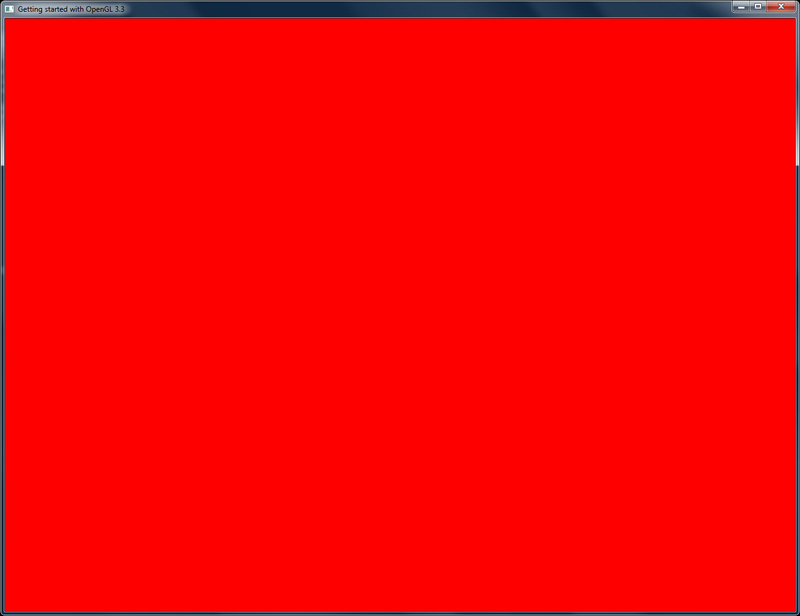## Designing a GLSL shader class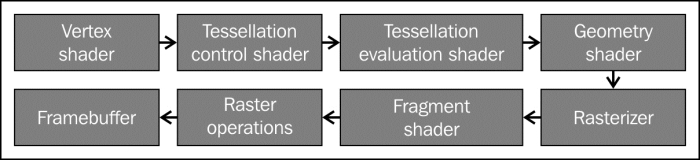Note that the tessellation control/evaluation shaders are only available in the hardware supporting OpenGL v4.0 and above. Since the steps involved in shader handling as well as compiling and attaching shaders for use in OpenGL applications are similar, we wrap these steps in a simple class we call `GLSLShader`.

The `GLSLShader` class is defined in the `GLSLShader.[h/cpp]` files. We first declare the constructor and destructor which initialize the member variables. The next three functions, `LoadFromString`, `LoadFromFile`, and `CreateAndLinkProgram` handle the shader compilation, linking, and program creation. The next two functions, `Use` and `UnUse` functions bind and unbind the program. Two `std::map` datastructures are used. They store the attribute's/uniform's name as the key and its location as the value. This is done to remove the redundant call to get the attribute's/uniform's location each frame or when the location is required to access the attribute/uniform. The next two functions, `AddAttribute` and `AddUniform` add the locations of the attribute and uniforms into their respective `std::map` (`_attributeList` and `_uniformLocationList`).

```class GLSLShader
{
public:
void Use();
void UnUse();
GLuint operator[](const string& attribute);
GLuint operator()(const string& uniform);

private:
GLuint  _program;
map<string,GLuint> _attributeList;
map<string,GLuint> _uniformLocationList;
};```

To make it convenient to access the attribute and uniform locations from their maps , we declare the two indexers. For attributes, we overload the square brackets ([]) whereas for uniforms, we overload the parenthesis operation (). Finally, we define a function `DeleteShaderProgram` for deletion of the shader program object. Following the function declarations are the member fields.

### How to do it…

In a typical shader application, the usage of the `GLSLShader` object is as follows:

1. Create the `GLSLShader` object either on stack (for example, `GLSLShader` shader;) or on the heap (for example, `GLSLShader* shader=new GLSLShader();`)

2. Call `LoadFromFile` on the `GLSLShader` object reference

3. Call `CreateAndLinkProgram` on the `GLSLShader` object reference

4. Call `Use` on the `GLSLShader` object reference to bind the shader object

5. Call `AddAttribute`/`AddUniform` to store locations of all of the shader's attributes and uniforms respectively

6. Call `UnUse` on the `GLSLShader` object reference to unbind the shader object

Note that the above steps are required at initialization only. We can set the values of the uniforms that remain constant throughout the execution of the application in the `Use`/`UnUse` block given above.

At the rendering step, we access uniform(s), if we have uniforms that change each frame (for example, the modelview matrices). We first bind the shader by calling the `GLSLShader::Use` function. We then set the uniform by calling the `glUniform{*}` function, invoke the rendering by calling the `glDraw{*}` function, and then unbind the shader (`GLSLShader::UnUse`). Note that the `glDraw{*}` call passes the attributes to the GPU.

### How it works…

In a typical OpenGL shader application, the shader specific functions and their sequence of execution are as follows:

```glCreateShader

Execution of the above four functions creates a shader object. After the shader object is created, a shader program object is created using the following set of functions in the following sequence:

```glCreateProgram
glGetProgramInfoLog```

### There's more…

In the `GLSLShader` class, the first four steps are handled in the `LoadFromString` function and the later four steps are handled by the `CreateAndLinkProgram` member function. After the shader program object has been created, we can set the program for execution on the GPU. This process is called shader binding. This is carried out by the `glUseProgram` function which is called through the `Use`/`UnUse` functions in the `GLSLShader` class.

To enable communication between the application and the shader, there are two different kinds of fields available in the shader. The first are the attributes which may change during shader execution across different shader stages. All per-vertex attributes fall in this category. The second are the uniforms which remain constant throughout the shader execution. Typical examples include the modelview matrix and the texture samplers.

In order to communicate with the shader program, the application must obtain the location of an attribute/uniform after the shader program is bound. The location identifies the attribute/uniform. In the `GLSLShader` class, for convenience, we store the locations of attributes and uniforms in two separate `std::map` objects.

For accessing any attribute/uniform location, we provide an indexer in the `GLSLShader` class. In cases where there is an error in the compilation or linking stage, the shader log is printed to the console. Say for example, our `GLSLshader` object is called `shader` and our `shader` contains a uniform called `MVP`. We can first add it to the map of `GLSLShader` by calling `shader.AddUniform("MVP")`. This function adds the uniform's location to the map. Then when we want to access the uniform, we directly call `shader("MVP")` and it returns the location of our uniform.

## Rendering a simple colored triangle using shaders

We will now put the `GLSLShader` class to use by implementing an application to render a simple colored triangle on screen.

For this recipe, we assume that the reader has created a new empty Win32 project with OpenGL 3.3 core profile as shown in the first recipe. The code for this recipe is in the `Chapter1/SimpleTriangle` directory.

### Tip

In all of the code samples in this book, you will see a macro `GL_CHECK_ERRORS` dispersed throughout. This macro checks the current error bit for any error which might be raised by passing invalid arguments to an OpenGL function, or when there is some problem with the OpenGL state machine. For any such error, this macro traps it and generates a debug assertion signifying that the OpenGL state machine has some error. In normal cases, no assertion should be raised, so adding this macro helps to identify errors. Since this macro calls `glGetError` inside a debug assert, it is stripped in the release build.

Now we will look at the different transformation stages through which a vertex goes, before it is finally rendered on screen. Initially, the vertex position is specified in what is called the object space. This space is the one in which the vertex location is specified for an object. We apply modeling transformation to the object space vertex position by multiplying it with an affine matrix (for example, a matrix for scaling, rotating, translating, and so on). This brings the object space vertex position into world space. Next, the world space positions are multiplied by the camera/viewing matrix which brings the position into view/eye/camera space. OpenGL stores the modeling and viewing transformations in a single (modelview) matrix.

The view space positions are then projected by using a projection transformation which brings the position into clip space. The clip space positions are then normalized to get the normalized device coordinates which have a canonical viewing volume (coordinates are [-1,-1,0] to [1,1,1] in x, y, and z coordinates respectively). Finally, the viewport transformation is applied which brings the vertex into window/screen space.

### How to do it…

Let us start this recipe using the following steps:

1. Define a vertex shader (`shaders/shader.vert`) to transform the object space vertex position to clip space.

```#version 330 core
layout(location = 0) in vec3 vVertex;
layout(location = 1) in vec3 vColor;
smooth out vec4 vSmoothColor;
uniform mat4 MVP;
void main()
{
vSmoothColor = vec4(vColor,1);
gl_Position = MVP*vec4(vVertex,1);
}```
2. Define a fragment shader (`shaders/shader.frag`) to output a smoothly interpolated color from the vertex shader to the frame buffer.

```#version 330 core
smooth in vec4 vSmoothColor;
layout(location=0) out vec4 vFragColor;
void main()
{
vFragColor = vSmoothColor;
}```
3. Load the two shaders using the `GLSLShader` class in the `OnInit()` function.

```shader.LoadFromFile(GL_VERTEX_SHADER, "shaders/shader.vert");
4. Create the geometry and topology. We will store the attributes together in an interleaved vertex format, that is, we will store the vertex attributes in a struct containing two attributes, position and color.

```vertices.color=glm::vec3(1,0,0);
vertices.color=glm::vec3(0,1,0);
vertices.color=glm::vec3(0,0,1);

vertices.position=glm::vec3(-1,-1,0);
vertices.position=glm::vec3(0,1,0);
vertices.position=glm::vec3(1,-1,0);

indices = 0;
indices = 1;
indices = 2;```
5. Store the geometry and topology in the buffer object(s). The stride parameter controls the number of bytes to jump to reach the next element of the same attribute. For the interleaved format, it is typically the size of our vertex struct in bytes, that is, `sizeof(Vertex)`.

```glGenVertexArrays(1, &vaoID);
glGenBuffers(1, &vboVerticesID);
glGenBuffers(1, &vboIndicesID);
glBindVertexArray(vaoID);
glBindBuffer (GL_ARRAY_BUFFER, vboVerticesID);
glBufferData (GL_ARRAY_BUFFER, sizeof(vertices), &vertices,               GL_STATIC_DRAW);
glVertexAttribPointer(shader["vColor"], 3, GL_FLOAT, GL_FALSE,stride, (const GLvoid*)offsetof(Vertex, color));

glBindBuffer(GL_ELEMENT_ARRAY_BUFFER, vboIndicesID);
glBufferData(GL_ELEMENT_ARRAY_BUFFER, sizeof(indices), &indices, GL_STATIC_DRAW);```
6. Set up the resize handler to set up the viewport and projection matrix.

```void OnResize(int w, int h) {
glViewport (0, 0, (GLsizei) w, (GLsizei) h);
P = glm::ortho(-1,1,-1,1);
}```
7. Set up the rendering code to bind the `GLSLShader` shader, pass the uniforms, and then draw the geometry.

```void OnRender() {
glClear(GL_COLOR_BUFFER_BIT|GL_DEPTH_BUFFER_BIT);
glDrawElements(GL_TRIANGLES, 3, GL_UNSIGNED_SHORT, 0);
glutSwapBuffers();
}```
8. Delete the shader and other OpenGL objects.

```void OnShutdown() {
glDeleteBuffers(1, &vboVerticesID);
glDeleteBuffers(1, &vboIndicesID);
glDeleteVertexArrays(1, &vaoID);
}```

### How it works…

For this simple example, we will only use a vertex shader (`shaders/shader.vert`) and a fragment shader (`shaders/shader.frag`). The first line in the shader signifies the GLSL version of the shader. Starting from OpenGL v3.0, the version specifiers correspond to the OpenGL version used. So for OpenGL v3.3, the GLSL version is 330. In addition, since we are interested in the core profile, we add another keyword following the version number to signify that we have a core profile shader.

Another important thing to note is the layout qualifier. This is used to bind a specific integral attribute index to a given per-vertex attribute. While we can give the attribute locations in any order, for all of the recipes in this book the attribute locations are specified starting from `0` for position, `1` for normals, `2` for texture coordinates, and so on. The layout location qualifier makes the `glBindAttribLocation` call redundant as the location index specified in the shader overrides any `glBindAttribLocation` call.

The vertex shader simply outputs the input per-vertex color to the output (`vSmoothColor`). Such attributes that are interpolated across shader stages are called varying attributes. It also calculates the clip space position by multiplying the per-vertex position (`vVertex`) with the combined modelview projection (MVP) matrix.

```vSmoothColor = vec4(vColor,1);
gl_Position = MVP*vec4(vVertex,1);```

### Tip

By prefixing `smooth` to the output attribute, we tell the GLSL shader to do smooth perspective-correct interpolation for the attribute to the next stage of the pipeline. The other qualifiers usable are `flat` and `noperspective`. When no qualifier is specified the default interpolation qualifier is `smooth`.

The fragment shader writes the input color (`vSmoothColor`) to the frame buffer output (`vFragColor`).

`vFragColor = vSmoothColor;`

### There's more…

In the simple triangle demo application code, we store the `GLSLShader` object reference in the global scope so that we can access it in any function we desire. We modify the `OnInit()` function by adding the following lines:

```shader.LoadFromFile(GL_VERTEX_SHADER, "shaders/shader.vert");

The first two lines create the GLSL shader of the given type by reading the contents of the file with the given filename. In all of the recipes in this book, the vertex shader files are stored with a `.vert` extension, the geometry shader files with a `.geom` extension, and the fragment shader files with a `.frag` extension. Next, the `GLSLShader::CreateAndLinkProgram` function is called to create the shader program from the shader object. Next, the program is bound and then the locations of attributes and uniforms are stored.

We pass two attributes per-vertex, that is vertex position and vertex color. In order to facilitate the data transfer to the GPU, we create a simple `Vertex` struct as follows:

```struct Vertex {
glm::vec3 position;
glm::vec3 color;
};
Vertex vertices;
GLushort indices;```

Next, we create an array of three vertices in the global scope. In addition, we store the triangle's vertex indices in the indices global array. Later we initialize these two arrays in the `OnInit()` function. The first vertex is assigned the red color, the second vertex is assigned the green color, and the third vertex is assigned the blue color.

```vertices.color=glm::vec3(1,0,0);
vertices.color=glm::vec3(0,1,0);
vertices.color=glm::vec3(0,0,1);

vertices.position=glm::vec3(-1,-1,0);
vertices.position=glm::vec3(0,1,0);
vertices.position=glm::vec3(1,-1,0);

indices = 0;
indices = 1;
indices = 2;```

Next, the vertex positions are given. The first vertex is assigned an object space position of (-1,-1, 0), the second vertex is assigned (0,1,0), and the third vertex is assigned (1,-1,0). For this simple demo, we use an orthographic projection for a view volume of (-1,1,-1,1). Finally, the three indices are given in a linear order.

In OpenGL v3.3 and above, we typically store the geometry information in buffer objects, which is a linear array of memory managed by the GPU. In order to facilitate the handling of buffer object(s) during rendering, we use a vertex array object (VAO). This object stores references to buffer objects that are bound after the VAO is bound. The advantage we get from using a VAO is that after the VAO is bound, we do not have to bind the buffer object(s).

In this demo, we declare three variables in global scope; `vaoID` for VAO handling, and `vboVerticesID` and `vboIndicesID` for buffer object handling. The VAO object is created by calling the `glGenVertexArrays` function. The buffer objects are generated using the `glGenBuffers` function. The first parameter for both of these functions is the total number of objects required, and the second parameter is the reference to where the object handle is stored. These functions are called in the `OnInit()` function.

```glGenVertexArrays(1, &vaoID);
glGenBuffers(1, &vboVerticesID);
glGenBuffers(1, &vboIndicesID);
glBindVertexArray(vaoID);```

After the VAO object is generated, we bind it to the current OpenGL context so that all successive calls affect the attached VAO object. After the VAO binding, we bind the buffer object storing vertices (`vboVerticesID`) using the `glBindBuffer` function to the `GL_ARRAY_BUFFER` binding. Next, we pass the data to the buffer object by using the `glBufferData` function. This function also needs the binding point, which is again `GL_ARRAY_BUFFER`. The second parameter is the size of the vertex array we will push to the GPU memory. The third parameter is the pointer to the start of the CPU memory. We pass the address of the vertices global array. The last parameter is the usage hint which tells the GPU that we are not going to modify the data often.

```glBindBuffer (GL_ARRAY_BUFFER, vboVerticesID);
glBufferData (GL_ARRAY_BUFFER, sizeof(vertices), &vertices, GL_STATIC_DRAW);```

The usage hints have two parts; the first part tells how frequently the data in the buffer object is modified. These can be `STATIC` (modified once only), `DYNAMIC` (modified occasionally), or `STREAM` (modified at every use). The second part is the way this data will be used. The possible values are `DRAW` (the data will be written but not read), `READ` (the data will be read only), and `COPY` (the data will be neither read nor written). Based on the two hints a qualifier is generated. For example, `GL_STATIC_DRAW` if the data will never be modified and `GL_DYNAMIC_DRAW` if the data will be modified occasionally. These hints allow the GPU and the driver to optimize the read/write access to this memory.

In the next few calls, we enable the vertex attributes. This function needs the location of the attribute, which we obtain by the `GLSLShader::operator[]`, passing it the name of the attribute whose location we require. We then call `glVertexAttributePointer` to tell the GPU how many elements there are and what is their type, whether the attribute is normalized, the stride (which means the total number of bytes to skip to reach the next element; for our case since the attributes are stored in a `Vertex` struct, the next element's stride is the size of our `Vertex` struct), and finally, the pointer to the attribute in the given array. The last parameter requires explanation in case we have interleaved attributes (as we have). The `offsetof` operator returns the offset in bytes, to the attribute in the given struct. Hence, the GPU knows how many bytes it needs to skip in order to access the next attribute of the given type. For the `vVertex` attribute, the last parameter is `0` since the next element is accessed immediately after the stride. For the second attribute `vColor`, it needs to hop 12 bytes before the next `vColor` attribute is obtained from the given vertices array.

```glEnableVertexAttribArray(shader["vVertex"]);
glVertexAttribPointer(shader["vColor"], 3, GL_FLOAT, GL_FALSE,stride, (const GLvoid*)offsetof(Vertex, color));```

The indices are pushed similarly using `glBindBuffer` and `glBufferData` but to a different binding point, that is, `GL_ELEMENT_ARRAY_BUFFER`. Apart from this change, the rest of the parameters are exactly the same as for the vertices data. The only difference being the buffer object, which for this case is `vboIndicesID`. In addition, the passed array to the `glBufferData` function is the indices array.

```glBindBuffer(GL_ELEMENT_ARRAY_BUFFER, vboIndicesID);
glBufferData(GL_ELEMENT_ARRAY_BUFFER, sizeof(indices), &indices, GL_STATIC_DRAW);```

To complement the object generation in the `OnInit()` function, we must provide the object deletion code. This is handled in the `OnShutdown()` function. We first delete the shader program by calling the `GLSLShader::DeleteShaderProgram` function. Next, we delete the two buffer objects (`vboVerticesID` and `vboIndicesID`) and finally we delete the vertex array object (`vaoID`).

```void OnShutdown() {
glDeleteBuffers(1, &vboVerticesID);
glDeleteBuffers(1, &vboIndicesID);
glDeleteVertexArrays(1, &vaoID);
}```

### Tip

We do a deletion of the shader program because our `GLSLShader` object is allocated globally and the destructor of this object will be called after the main function exits. Therefore, if we do not delete the object in this function, the shader program will not be deleted and we will have a graphics memory leak.

The rendering code of the simple triangle demo is as follows:

```void OnRender() {
glClear(GL_COLOR_BUFFER_BIT|GL_DEPTH_BUFFER_BIT);
glDrawElements(GL_TRIANGLES, 3, GL_UNSIGNED_SHORT, 0);
glutSwapBuffers();
}```

The rendering code first clears the color and depth buffer and binds the shader program by calling the `GLSLShader::Use()` function. It then passes the combined modelview and projection matrix to the GPU by invoking the `glUniformMatrix4fv` function. The first parameter is the location of the uniform which we obtain from the `GLSLShader::operator()` function, by passing it the name of the uniform whose location we need. The second parameter is the total number of matrices we wish to pass. The third parameter is a Boolean signifying if the matrix needs to be transposed, and the final parameter is the float pointer to the matrix object. Here we use the `glm::value_ptr` function to get the float pointer from the matrix object. Note that the OpenGL matrices are concatenated right to left since it follows a right handed coordinate system in a column major layout. Hence we keep the projection matrix on the left and the modelview matrix on the right. For this simple example, the modelview matrix (`MV`) is set as the identity matrix.

After this function, the `glDrawElements` call is made. Since we have left our VAO object (`vaoID`) bound, we pass `0` to the final parameter of this function. This tells the GPU to use the references of the `GL_ELEMENT_ARRAY_BUFFER` and `GL_ARRAY_BUFFER` binding points of the bound VAO. Thus we do not need to explicitly bind the `vboVerticesID` and `vboIndicesID` buffer objects again. After this call, we unbind the shader program by calling the `GLSLShader::UnUse()` function. Finally, we call the `glutSwapBuffer` function to show the back buffer on screen. After compiling and running, we get the output as shown in the following figure: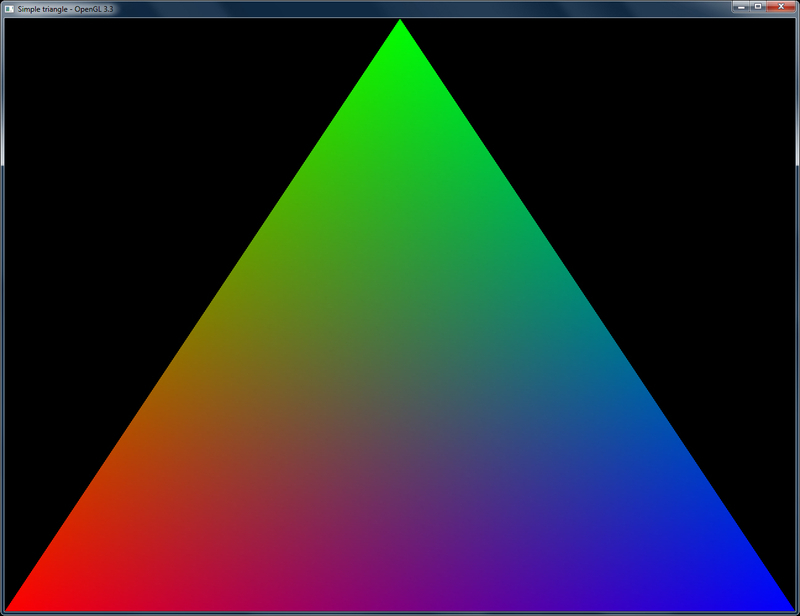Learn modern 3D graphics programming by Jason L. McKesson at http://www.arcsynthesis.org/gltut/Basics/Basics.html.

## Doing a ripple mesh deformer using the vertex shader

In this recipe, we will deform a planar mesh using the vertex shader. We know that the vertex shader is responsible for outputting the clip space position of the given object space vertex. In between this conversion, we can apply the modeling transformation to transform the given object space vertex to world space position.

For this recipe, we assume that the reader knows how to set up a simple triangle on screen using a vertex and fragment shader as detailed in the previous recipe. The code for this recipe is in the `Chapter1\RippleDeformer` directory.

### How to do it…

We can implement a ripple shader using the following steps:

1. Define the vertex shader that deforms the object space vertex position.

```#version 330 core
layout(location=0) in vec3 vVertex;
uniform mat4 MVP;
uniform float time;
const float amplitude = 0.125;
const float frequency = 4;
const float PI = 3.14159;
void main()
{
float distance = length(vVertex);
float y = amplitude*sin(-PI*distance*frequency+time);
gl_Position = MVP*vec4(vVertex.x, y, vVertex.z,1);
}```
2. Define a fragment shader that simply outputs a constant color.

```#version 330 core
layout(location=0) out vec4 vFragColor;
void main()
{
vFragColor = vec4(1,1,1,1);
}```
3. Load the two shaders using the `GLSLShader` class in the `OnInit()` function.

```shader.LoadFromFile(GL_VERTEX_SHADER, "shaders/shader.vert");
4. Create the geometry and topology.

```int count = 0;
int i=0, j=0;
for( j=0;j<=NUM_Z;j++) {
for( i=0;i<=NUM_X;i++) {
vertices[count++] = glm::vec3( ((float(i)/(NUM_X-1)) *2-1)* HALF_SIZE_X, 0, ((float(j)/(NUM_Z-1))*2-1)*HALF_SIZE_Z);
}
}
GLushort* id=&indices;
for (i = 0; i < NUM_Z; i++) {
for (j = 0; j < NUM_X; j++) {
int i0 = i * (NUM_X+1) + j;
int i1 = i0 + 1;
int i2 = i0 + (NUM_X+1);
int i3 = i2 + 1;
if ((j+i)%2) {
*id++ = i0; *id++ = i2; *id++ = i1;
*id++ = i1; *id++ = i2; *id++ = i3;
} else {
*id++ = i0; *id++ = i2; *id++ = i3;
*id++ = i0; *id++ = i3; *id++ = i1;
}
}
}```
5. Store the geometry and topology in the buffer object(s).

```glGenVertexArrays(1, &vaoID);
glGenBuffers(1, &vboVerticesID);
glGenBuffers(1, &vboIndicesID);
glBindVertexArray(vaoID);
glBindBuffer (GL_ARRAY_BUFFER, vboVerticesID);
glBufferData (GL_ARRAY_BUFFER, sizeof(vertices), &vertices, GL_STATIC_DRAW);
glBindBuffer(GL_ELEMENT_ARRAY_BUFFER, vboIndicesID);
glBufferData(GL_ELEMENT_ARRAY_BUFFER, sizeof(indices), &indices, GL_STATIC_DRAW);```
6. Set up the perspective projection matrix in the resize handler.

`P = glm::perspective(45.0f, (GLfloat)w/h, 1.f, 1000.f);`
7. Set up the rendering code to bind the `GLSLShader` shader, pass the uniforms and then draw the geometry.

```void OnRender() {
time = glutGet(GLUT_ELAPSED_TIME)/1000.0f * SPEED;
glm::mat4 T=glm::translate(glm::mat4(1.0f), glm::vec3(0.0f, 0.0f, dist));
glm::mat4 Rx= glm::rotate(T,  rX, glm::vec3(1.0f, 0.0f, 0.0f));
glm::mat4 MV= glm::rotate(Rx, rY, glm::vec3(0.0f, 1.0f, 0.0f));
glm::mat4 MVP= P*MV;
glDrawElements(GL_TRIANGLES,TOTAL_INDICES,GL_UNSIGNED_SHORT,0);
glutSwapBuffers();
}```
8. Delete the shader and other OpenGL objects.

```void OnShutdown() {
glDeleteBuffers(1, &vboVerticesID);
glDeleteBuffers(1, &vboIndicesID);
glDeleteVertexArrays(1, &vaoID);
}```

### How it works…

In this recipe, the only attribute passed in is the per-vertex position (`vVertex`). There are two uniforms: the combined modelview projection matrix (`MVP`) and the current time (`time`). We will use the `time` uniform to allow progression of the deformer so we can observe the ripple movement. After these declarations are three constants, namely `amplitude` (which controls how much the ripple moves up and down from the zero base line), `frequency` (which controls the total number of waves), and `PI` (a constant used in the wave formula). Note that we could have replaced the constants with uniforms and had them modified from the application code.

Now the real work is carried out in the main function. We first find the distance of the given vertex from the origin. Here we use the `length` built-in GLSL function. We then create a simple sinusoid. We know that a general sine wave can be given using the following function: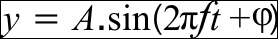Here, `A` is the wave amplitude, `f` is the frequency, `t` is the time, and `φ` is the phase. In order to get our ripple to start from the origin, we modify the function to the following: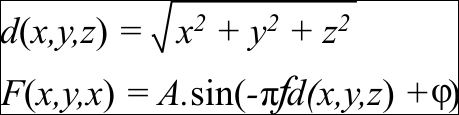In our formula, we first find the distance (`d`) of the vertex from the origin by using the Euclidean distance formula. This is given to us by the `length` built-in GLSL function. Next, we input the distance into the `sin` function multiplying the distance by the frequency (`f`) and (`π`). In our vertex shader, we replace the phase (`φ`) with time.

```#version 330 core
layout(location=0) in vec3 vVertex;
uniform mat4 MVP;
uniform float time;
const float amplitude = 0.125;
const float frequency = 4;
const float PI = 3.14159;
void main()
{
float distance = length(vVertex);
float y = amplitude*sin(-PI*distance*frequency+time);
gl_Position = MVP*vec4(vVertex.x, y, vVertex.z,1);
}```

After calculating the new `y` value, we multiply the new vertex position with the combined modelview projection matrix (`MVP`). The fragment shader simply outputs a constant color (in this case white color, `vec4(1,1,1,1)`).

```#version 330 core
layout(location=0) out vec4 vFragColor;
void main()
{
vFragColor = vec4(1,1,1,1);
}```

### There's more

Similar to the previous recipe, we declare the `GLSLShader` object in the global scope to allow maximum visibility. Next, we initialize the `GLSLShader` object in the `OnInit()` function.

```shader.LoadFromFile(GL_VERTEX_SHADER, "shaders/shader.vert");

The only difference in this recipe is the addition of an additional uniform (`time`).

We generate a simple 3D planar grid in the XZ plane. The geometry is stored in the vertices global array. The total number of vertices on the X axis is stored in a global constant `NUM_X`, whereas the total number of vertices on the Z axis is stored in another global constant `NUM_Z`. The size of the planar grid in world space is stored in two global constants, `SIZE_X` and `SIZE_Z`, and half of these values are stored in the `HALF_SIZE_X` and `HALF_SIZE_Z` global constants. Using these constants, we can change the mesh resolution and world space size.

The loop simply iterates `(NUM_X+1)*(NUM_Z+1)` times and remaps the current vertex index first into the `0` to `1` range and then into the `-1` to `1` range, and finally multiplies it by the `HALF_SIZE_X` and `HALF_SIZE_Z` constants to get the range from `–HALF_SIZE_X` to `HALF_SIZE_X` and `–HALF_SIZE_Z` to `HALF_SIZE_Z`.

The topology of the mesh is stored in the indices global array. While there are several ways to generate the mesh topology, we will look at two common ways. The first method keeps the same triangulation for all of the mesh quads as shown in the following screenshot: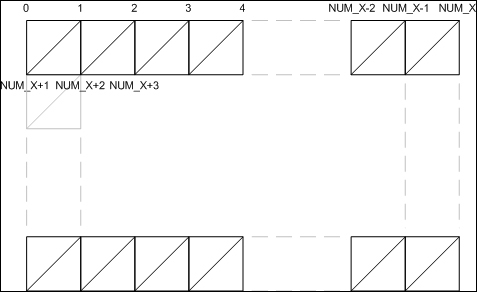This sort of topology can be generated using the following code:

```GLushort* id=&indices;
for (i = 0; i < NUM_Z; i++) {
for (j = 0; j < NUM_X; j++) {
int i0 = i * (NUM_X+1) + j;
int i1 = i0 + 1;
int i2 = i0 + (NUM_X+1);
int i3 = i2 + 1;
*id++ = i0; *id++ = i2; *id++ = i1;
*id++ = i1; *id++ = i2; *id++ = i3;
}
}```

The second method alternates the triangulation at even and odd iterations resulting in a better looking mesh as shown in the following screenshot: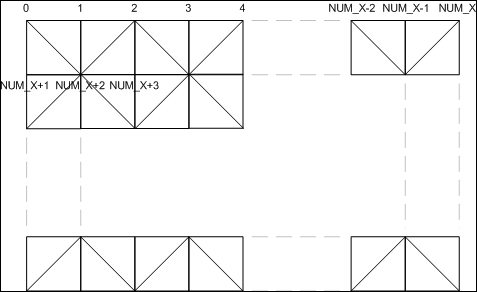In order to alternate the triangle directions and maintain their winding order, we take two different combinations, one for an even iteration and second for an odd iteration. This can be achieved using the following code:

```GLushort* id=&indices;
for (i = 0; i < NUM_Z; i++) {
for (j = 0; j < NUM_X; j++) {
int i0 = i * (NUM_X+1) + j;
int i1 = i0 + 1;
int i2 = i0 + (NUM_X+1);
int i3 = i2 + 1;
if ((j+i)%2) {
*id++ = i0; *id++ = i2; *id++ = i1;
*id++ = i1; *id++ = i2; *id++ = i3;
} else {
*id++ = i0; *id++ = i2; *id++ = i3;
*id++ = i0; *id++ = i3; *id++ = i1;
}
}
}```

After filling the vertices and indices arrays, we push this data to the GPU memory. We first create a vertex array object (`vaoID`) and two buffer objects, the `GL_ARRAY_BUFFER` binding for vertices and the `GL_ELEMENT_ARRAY_BUFFER` binding for the indices array. These calls are exactly the same as in the previous recipe. The only difference is that now we only have a single per-vertex attribute, that is, the vertex position (`vVertex`). The `OnShutdown()` function is also unchanged as in the previous recipe.

The rendering code is slightly changed. We first get the current elapsed time from freeglut so that we can move the ripple deformer in time. Next, we clear the color and depth buffers. After this, we set up the modelview matrix. This is carried out by using the matrix transformation functions provided by the `glm` library.

```glm::mat4 T=glm::translate(glm::mat4(1.0f),
glm::vec3(0.0f, 0.0f, dist));
glm::mat4 Rx= glm::rotate(T,  rX, glm::vec3(1.0f, 0.0f, 0.0f));
glm::mat4 MV= glm::rotate(Rx, rY, glm::vec3(0.0f, 1.0f,  0.0f));
glm::mat4 MVP= P*MV;```

Note that the matrix multiplication in `glm` follows from right to left. So the order in which we generate the transformations will be applied in the reverse order. In our case the combined modelview matrix will be calculated as `MV = (T*(Rx*Ry))`. The translation amount, `dist`, and the rotation values, `rX` and `rY`, are calculated in the mouse input functions based on the user's input.

After calculating the modelview matrix, the combined modelview projection matrix (`MVP`) is calculated. The projection matrix (`P`) is calculated in the `OnResize()` handler. In this case, the perspective projection matrix is used with four parameters, the vertical fov, the aspect ratio, and the near and far clip plane distances. The `GLSLShader` object is bound and then the two uniforms, `MVP` and `time` are passed to the shader program. The attributes are then transferred using the `glDrawElements` call as we saw in the previous recipe. The `GLSLShader` object is then unbound and finally, the back buffer is swapped.

In the ripple deformer main function, we attach two new callbacks; `glutMouseFunc` handled by the `OnMouseDown` function and `glutMotionFunc` handled by the `OnMouseMove` function. These functions are defined as follows:

```void OnMouseDown(int button, int s, int x, int y) {
if (s == GLUT_DOWN)  {
oldX = x;
oldY = y;
}
if(button == GLUT_MIDDLE_BUTTON)
state = 0;
else
state = 1;
}```

This function is called whenever the mouse is clicked in our application window. The first parameter is for the button which was pressed (`GLUT_LEFT_BUTTON` for the left mouse button, `GLUT_MIDDLE_BUTTON` for the middle mouse button, and `GLUT_RIGHT_BUTTON` for the right mouse button). The second parameter is the state which can be either `GLUT_DOWN` or `GLUT_UP`. The last two parameters are the `x` and `y` screen location of the mouse click. In this simple example, we store the mouse click location and then set a state variable when the middle mouse button is pressed.

The `OnMouseMove` function is defined as follows:

```void OnMouseMove(int x, int y) {
if (state == 0)
dist *= (1 + (y - oldY)/60.0f);
else {
rY += (x - oldX)/5.0f;
rX += (y - oldY)/5.0f;
}
oldX = x; oldY = y;
glutPostRedisplay();
}```

The `OnMouseMove` function has only two parameters, the `x` and `y` screen location where the mouse currently is. The mouse move event is raised whenever the mouse enters and moves in the application window. Based on the state set in the `OnMouseDown` function, we calculate the zoom amount (`dist`) if the middle mouse button is pressed. Otherwise, we calculate the two rotation amounts (`rX` and `rY`). Next, we update the `oldX` and `oldY` positions for the next event. Finally we request the freeglut framework to repaint our application window by calling `glutPostRedisplay()` function. This call sends the repaint event which re-renders our scene.

In order to make it easy for us to see the deformation, we enable wireframe rendering by calling the `glPolygonMode(GL_FRONT_AND_BACK, GL_LINE)` function in the `OnInit()` function.

### Tip

There are two things to be careful about with the `glPolygonMode` function. Firstly, the first parameter can only be `GL_FRONT_AND_BACK` in the core profile. Secondly, make sure that the second parameter is named `GL_LINE` instead of `GL_LINES` which is used with the `glDraw*` functions. To disable the wireframe rendering and return to the default fill rendering, change the second parameter from `GL_LINE` to `GL_FILL`.

Running the demo code shows a ripple deformer propagating the deformation in a mesh grid as shown in the following screenshot. Hopefully, this recipe should have cleared how to use vertex shaders, especially for doing per-vertex transformations.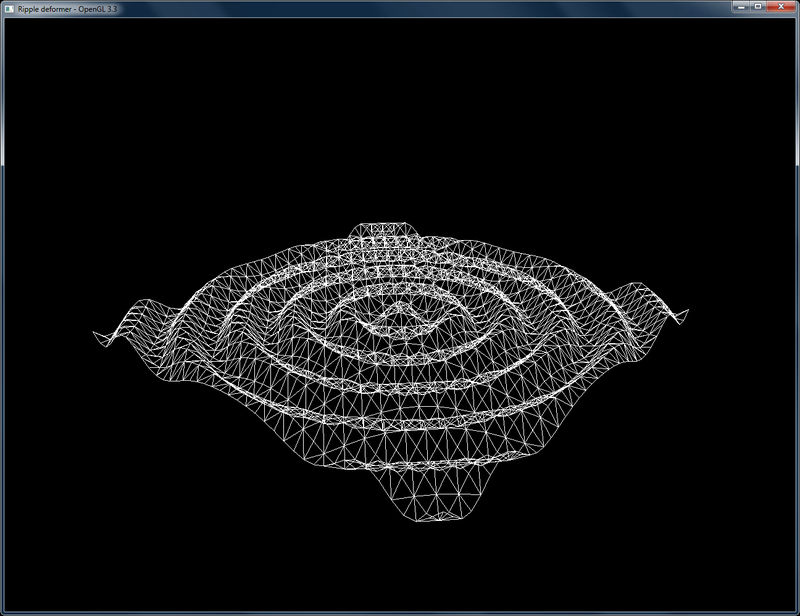## Dynamically subdividing a plane using the geometry shader

After the vertex shader, the next programmable stage in the OpenGL v3.3 graphics pipeline is the geometry shader. This shader contains inputs from the vertex shader stage. We can either feed these unmodified to the next shader stage or we can add/omit/modify vertices and primitives as desired. One thing that the vertex shaders lack is the availability of the other vertices of the primitive. Geometry shaders have information of all on the vertices of a single primitive.

The advantage with geometry shaders is that we can add/remove primitives on the fly. Moreover it is easier to get all vertices of a single primitive, unlike in the vertex shader, which has information on a single vertex only. The main drawback of geometry shaders is the limit on the number of new vertices we can generate, which is dependent on the hardware. Another disadvantage is the limited availability of the surrounding primitives.

In this recipe, we will dynamically subdivide a planar mesh using the geometry shader.

This recipe assumes that the reader knows how to render a simple triangle using vertex and fragment shaders using the OpenGL v3.3 core profile. We render four planar meshes in this recipe which are placed next to each other to create a bigger planar mesh. Each of these meshes is subdivided using the same geometry shader. The code for this recipe is located in the `Chapter1\SubdivisionGeometryShader` directory.

### How to do it…

We can implement the geometry shader using the following steps:

1. Define a vertex shader (`shaders/shader.vert`) which outputs object space vertex positions directly.

```#version 330 core
layout(location=0) in vec3 vVertex;
void main() {
gl_Position =  vec4(vVertex, 1);
}```
2. Define a geometry shader (`shaders/shader.geom`) which performs the subdivision of the quad. The shader is explained in the next section.

```#version 330 core
layout (triangles) in;
layout (triangle_strip, max_vertices=256) out;
uniform int sub_divisions;
uniform mat4 MVP;
void main() {
vec4 v0 = gl_in.gl_Position;
vec4 v1 = gl_in.gl_Position;
vec4 v2 = gl_in.gl_Position;
float dx = abs(v0.x-v2.x)/sub_divisions;
float dz = abs(v0.z-v1.z)/sub_divisions;
float x=v0.x;
float z=v0.z;
for(int j=0;j<sub_divisions*sub_divisions;j++) {
gl_Position =  MVP * vec4(x,0,z,1);
EmitVertex();
gl_Position =  MVP * vec4(x,0,z+dz,1);
EmitVertex();
gl_Position =  MVP * vec4(x+dx,0,z,1);
EmitVertex();
gl_Position =  MVP * vec4(x+dx,0,z+dz,1);
EmitVertex();
EndPrimitive();
x+=dx;
if((j+1) %sub_divisions == 0) {
x=v0.x;
z+=dz;
}
}
}```
3. Define a fragment shader (`shaders/shader.frag`) that simply outputs a constant color.

```#version 330 core
layout(location=0) out vec4 vFragColor;
void main() {
vFragColor = vec4(1,1,1,1);
}```
4. Load the shaders using the GLSLShader class in the `OnInit()` function.

```shader.LoadFromFile(GL_VERTEX_SHADER, "shaders/shader.vert");
5. Create the geometry and topology.

```vertices = glm::vec3(-5,0,-5);
vertices = glm::vec3(-5,0,5);
vertices = glm::vec3(5,0,5);
vertices = glm::vec3(5,0,-5);
GLushort* id=&indices;

*id++ = 0;
*id++ = 1;
*id++ = 2;
*id++ = 0;
*id++ = 2;
*id++ = 3;```
6. Store the geometry and topology in the buffer object(s). Also enable the line display mode.

```glGenVertexArrays(1, &vaoID);
glGenBuffers(1, &vboVerticesID);
glGenBuffers(1, &vboIndicesID);
glBindVertexArray(vaoID);
glBindBuffer (GL_ARRAY_BUFFER, vboVerticesID);
glBufferData (GL_ARRAY_BUFFER, sizeof(vertices), &vertices, GL_STATIC_DRAW);
glBindBuffer(GL_ELEMENT_ARRAY_BUFFER, vboIndicesID);
glBufferData(GL_ELEMENT_ARRAY_BUFFER, sizeof(indices), &indices, GL_STATIC_DRAW);
glPolygonMode(GL_FRONT_AND_BACK, GL_LINE);```
7. Set up the rendering code to bind the `GLSLShader` shader, pass the uniforms and then draw the geometry.

```void OnRender() {
glClear(GL_COLOR_BUFFER_BIT|GL_DEPTH_BUFFER_BIT);
glm::mat4 T = glm::translate( glm::mat4(1.0f), glm::vec3(0.0f,0.0f, dist));
glm::mat4 Rx=glm::rotate(T,rX,glm::vec3(1.0f, 0.0f, 0.0f));
glm::mat4 MV=glm::rotate(Rx,rY, glm::vec3(0.0f,1.0f,0.0f));
MV=glm::translate(MV, glm::vec3(-5,0,-5));
glDrawElements(GL_TRIANGLES, 6, GL_UNSIGNED_SHORT, 0);

MV=glm::translate(MV, glm::vec3(10,0,0));
glDrawElements(GL_TRIANGLES, 6, GL_UNSIGNED_SHORT, 0);

MV=glm::translate(MV, glm::vec3(0,0,10));
glDrawElements(GL_TRIANGLES, 6, GL_UNSIGNED_SHORT, 0);

MV=glm::translate(MV, glm::vec3(-10,0,0));
glDrawElements(GL_TRIANGLES, 6, GL_UNSIGNED_SHORT, 0);
glutSwapBuffers();
}```
8. Delete the shader and other OpenGL objects.

```void OnShutdown() {
glDeleteBuffers(1, &vboVerticesID);
glDeleteBuffers(1, &vboIndicesID);
glDeleteVertexArrays(1, &vaoID);
cout<<"Shutdown successfull"<<endl;
}```

### How it works…

```#version 330 core
layout (triangles) in;
layout (triangle_strip, max_vertices=256) out;```

The first line signifies the GLSL version of the shader. The next two lines are important as they tell the shader processor about the input and output primitives of our geometry shader. In this case, the input will be `triangles` and the output will be a `triangle_strip`.

In addition, we also need to give the maximum number of output vertices from this geometry shader. This is a hardware specific number. For the hardware used in this development, the `max_vertices` value is found to be `256`. This information can be obtained by querying the `GL_MAX_GEOMETRY_OUTPUT_VERTICES` field and it is dependent on the primitive type used and the number of attributes stored per-vertex.

```uniform int sub_divisions;
uniform mat4 MVP;```

Next, we declare two uniforms, the total number of subdivisions desired (`sub_divisions`) and the combined modelview projection matrix (`MVP`).

```void main() {
vec4 v0 = gl_in.gl_Position;
vec4 v1 = gl_in.gl_Position;
vec4 v2 = gl_in.gl_Position;```

The bulk of the work takes place in the main entry point function. For each triangle pushed from the application, the geometry shader is run once. Thus, for each triangle, the positions of its vertices are obtained from the `gl_Position` attribute which is stored in the built-in `gl_in` array. All other attributes are input as an array in the geometry shader. We store the input positions in local variable `v0`, `v1`, and `v2`.

Next, we calculate the size of the smallest quad for the given subdivision based on the size of the given base triangle and the total number of subdivisions required.

```float dx = abs(v0.x-v2.x)/sub_divisions;
float dz = abs(v0.z-v1.z)/sub_divisions;
float x=v0.x;
float z=v0.z;
for(int j=0;j<sub_divisions*sub_divisions;j++) {
gl_Position =  MVP * vec4(x,   0,   z,1);  EmitVertex();
gl_Position =  MVP * vec4(x,   0,z+dz,1);  EmitVertex();
gl_Position =  MVP * vec4(x+dx,0,   z,1);  EmitVertex();
gl_Position =  MVP * vec4(x+dx,0,z+dz,1);  EmitVertex();
EndPrimitive();
x+=dx;
if((j+1) % sub_divisions == 0) {
x=v0.x;
z+=dz;
}
}
}```

We start from the first vertex. We store the `x` and `z` values of this vertex in local variables. Next, we iterate `N*N` times, where `N` is the total number of subdivisions required. For example, if we need to subdivide the mesh three times on both axes, the loop will run nine times, which is the total number of quads. After calculating the positions of the four vertices, they are emitted by calling `EmitVertex()`. This function emits the current values of output variables to the current output primitive on the primitive stream. Next, the `EndPrimitive()` call is issued to signify that we have emitted the four vertices of `triangle_strip`.

After these calculations, the local variable `x` is incremented by `dx` amount. If we are at an iteration that is a multiple of `sub_divisions`, we reset variable `x` to the `x` value of the first vertex while incrementing the local variable `z`.

The fragment shader outputs a constant color (white: `vec4(1,1,1,1)`).

### There's more…

The application code is similar to the last recipes. We have an additional shader (`shaders/shader.geom`), which is our geometry shader that is loaded from file.

```shader.LoadFromFile(GL_VERTEX_SHADER, "shaders/shader.vert");

The notable additions are highlighted, which include the new geometry shader and an additional uniform for the total subdivisions desired (`sub_divisions`). We initialize this uniform at initialization. The buffer object handling is similar to the simple triangle recipe. The other difference is in the rendering function where there are some additional modeling transformations (translations) after the viewing transformation.

The `OnRender()` function starts by clearing the color and depth buffers. It then calculates the viewing transformation as in the previous recipe.

```void OnRender() {
glClear(GL_COLOR_BUFFER_BIT|GL_DEPTH_BUFFER_BIT);
glm::mat4 T = glm::translate( glm::mat4(1.0f), glm::vec3(0.0f,0.0f, dist));
glm::mat4 Rx=glm::rotate(T,rX,glm::vec3(1.0f, 0.0f, 0.0f));
glm::mat4 MV=glm::rotate(Rx,rY, glm::vec3(0.0f,1.0f,0.0f));
MV=glm::translate(MV, glm::vec3(-5,0,-5));
```

Since our planer mesh geometry is positioned at origin going from -5 to 5 on the X and Z axes, we have to place them in the appropriate place by translating them, otherwise they would overlay each other.

Next, we first bind the shader program. Then we pass the shader uniforms which include the `sub_divisions` uniform and the combined modelview projection matrix (`MVP`) uniform. Then we pass the attributes by issuing a call to the `glDrawElements` function. We then add the relative translation for each instance to get a new modelview matrix for the next draw call. This is repeated three times to get all four planar meshes placed properly in the world space.

In this recipe, we handle keyboard input to allow the user to change the subdivision level dynamically. We first attach our keyboard event handler (`OnKey`) to `glutKeyboardFunc`. The keyboard event handler is defined as follows:

```void OnKey(unsigned char key, int x, int y) {
switch(key) {
case ',':  sub_divisions--; break;
case '.':  sub_divisions++; break;
}
sub_divisions = max(1,min(8, sub_divisions));
glutPostRedisplay();
}```

We can change the subdivision levels by pressing the , and . keys. We then check to make sure that the subdivisions are within the allowed limit. Finally, we request the freeglut function, `glutPostRedisplay()`, to repaint the window to show the new mesh. Compiling and running the demo code displays four planar meshes. Pressing the , key decreases the subdivision level and the . key increases the subdivision level. The output from the subdivision geometry shader showing multiple subdivision levels is displayed in the following screenshot: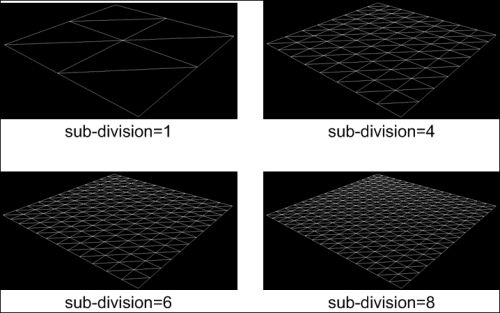You can view the Geometry shader tutorial part 1 and 2 at Geeks3D:

## Dynamically subdividing a plane using the geometry shader with instanced rendering

In order to avoid pushing the same data multiple times, we can exploit the instanced rendering functions. We will now see how we can omit the multiple `glDrawElements` calls in the previous recipe with a single `glDrawElementsInstanced` call.

Before doing this, we assume that the reader knows how to use the geometry shader in the OpenGL 3.3 core profile. The code for this recipe is in the `Chapter1\SubdivisionGeometryShader_Instanced` directory.

### How to do it…

Converting the previous recipe to use instanced rendering requires the following steps:

1. Change the vertex shader to handle the instance modeling matrix and output world space positions (`shaders/shader.vert`).

```#version 330 core
layout(location=0) in vec3 vVertex;
uniform mat4 M;
void main()
{
gl_Position =  M[gl_InstanceID]*vec4(vVertex, 1);
}```
2. Change the geometry shader to replace the `MVP` matrix with the `PV` matrix (`shaders/shader.geom`).

```#version 330 core
layout (triangles) in;
layout (triangle_strip, max_vertices=256) out;
uniform int sub_divisions;
uniform mat4 PV;

void main()
{
vec4 v0 = gl_in.gl_Position;
vec4 v1 = gl_in.gl_Position;
vec4 v2 = gl_in.gl_Position;
float dx = abs(v0.x-v2.x)/sub_divisions;
float dz = abs(v0.z-v1.z)/sub_divisions;
float x=v0.x;
float z=v0.z;
for(int j=0;j<sub_divisions*sub_divisions;j++) {
gl_Position =  PV * vec4(x,0,z,1);        EmitVertex();
gl_Position =  PV * vec4(x,0,z+dz,1);     EmitVertex();
gl_Position =  PV * vec4(x+dx,0,z,1);     EmitVertex();
gl_Position =  PV * vec4(x+dx,0,z+dz,1);  EmitVertex();
EndPrimitive();
x+=dx;
if((j+1) %sub_divisions == 0) {
x=v0.x;
z+=dz;
}
}
}```
3. Initialize the per-instance model matrices (`M`).

```void OnInit() {
//set the instance modeling matrix
M = glm::translate(glm::mat4(1), glm::vec3(-5,0,-5));
M = glm::translate(M, glm::vec3(10,0,0));
M = glm::translate(M, glm::vec3(0,0,10));
M = glm::translate(M, glm::vec3(-10,0,0));
..
4. Render instances using the `glDrawElementInstanced` call.

```void OnRender() {
glClear(GL_COLOR_BUFFER_BIT|GL_DEPTH_BUFFER_BIT);
glm::mat4 T =glm::translate(glm::mat4(1.0f), glm::vec3(0.0f, 0.0f, dist));
glm::mat4 Rx=glm::rotate(T,rX,glm::vec3(1.0f, 0.0f, 0.0f));
glm::mat4 V =glm::rotate(Rx,rY,glm::vec3(0.0f, 1.0f,0.0f));
glm::mat4 PV = P*V;

glDrawElementsInstanced(GL_TRIANGLES, 6, GL_UNSIGNED_SHORT, 0, 4);
glutSwapBuffers();
}```

### How it works…

First, we need to store the model matrix for each instance separately. Since we have four instances, we store a uniform array of four elements (`M`). Second, we multiply the per-vertex position (`vVertex`) with the model matrix for the current instance (`M[gl_InstanceID]`).

### Tip

Note that the `gl_InstanceID` built-in attribute will be filled with the index of each instance automatically at the time of the `glDrawElementsInstanced` call. Also note that this built-in attribute is only accessible in the vertex shader.

The `MVP` matrix is omitted from the geometry shader since now the input vertex positions are in world space. So we only need to multiply them with the combined view projection (`PV`) matrix. On the application side, the `MV` matrix is removed. Instead, we store the model matrix array for all four instances (`glm::mat4 M`). The values of these matrices are initialized in the `OnInit()` function as follows:

```M = glm::translate(glm::mat4(1), glm::vec3(-5,0,-5));
M = glm::translate(M, glm::vec3(10,0,0));
M = glm::translate(M, glm::vec3(0,0,10));
M = glm::translate(M, glm::vec3(-10,0,0));```

The rendering function, `OnRender()`, creates the combined view projection matrix (`PV`) and then calls `glDrawElementsInsntanced`. The first four parameters are similar to the `glDrawElements` function. The final parameter is the total number of instances desired. Instanced rendering is an efficient mechanism for rendering identical geometry whereby the `GL_ARRAY_BUFFER` and `GL_ELEMENT_ARRAY_BUFFER` bindings are shared between instances allowing the GPU to do efficient resource access and sharing.

```void OnRender() {
glClear(GL_COLOR_BUFFER_BIT|GL_DEPTH_BUFFER_BIT);
glm::mat4 T = glm::translate(glm::mat4(1.0f),glm::vec3(0.0f, 0.0f, dist));
glm::mat4 Rx = glm::rotate(T,  rX, glm::vec3(1.0f, 0.0f, 0.0f));
glm::mat4 V = glm::rotate(Rx, rY, glm::vec3(0.0f, 1.0f, 0.0f));
glm::mat4 PV = P*V;
glDrawElementsInstanced(GL_TRIANGLES,6,GL_UNSIGNED_SHORT,0, 4);
glutSwapBuffers();
}```

There is always a limit on the maximum number of matrices one can output from the vertex shader and this has some performance implications as well. Some performance improvements can be obtained by replacing the matrix storage with translation and scaling vectors, and an orientation quaternion which can then be converted on the fly into a matrix in the shader.

The official OpenGL wiki can be found at http://www.opengl.org/wiki/Built-in_Variable_%28GLSL%29.

An instance rendering tutorial from OGLDev can be found at http://ogldev.atspace.co.uk/www/tutorial33/tutorial33.html.

## Drawing a 2D image in a window using the fragment shader and the SOIL image loading library

We will wrap up this chapter with a recipe for creating a simple image viewer in the OpenGL v3.3 core profile using the `SOIL` image loading library.

After setting up the Visual Studio environment, we can now work with the SOIL library. The code for this recipe is in the `Chapter1/ImageLoader` directory.

### How to do it…

Let us now implement the image loader by following these steps:

1. Load the image using the `SOIL` library. Since the loaded image from `SOIL` is inverted vertically, we flip the image on the Y axis.

```int texture_width = 0, texture_height = 0, channels=0;
if(pData == NULL) {
exit(EXIT_FAILURE);
}
int i,j;
for( j = 0; j*2 < texture_height; ++j )
{
int index1 = j * texture_width * channels;
int index2 = (texture_height - 1 - j) * texture_width * channels;
for( i = texture_width * channels; i > 0; --i )
{
GLubyte temp = pData[index1];
pData[index1] = pData[index2];
pData[index2] = temp;
++index1;
++index2;
}
}```
2. Set up the OpenGL texture object and free the data allocated by the `SOIL` library.

```glGenTextures(1, &textureID);
glActiveTexture(GL_TEXTURE0);
glBindTexture(GL_TEXTURE_2D, textureID);
glTexParameteri(GL_TEXTURE_2D, GL_TEXTURE_MIN_FILTER,GL_LINEAR);
glTexParameteri(GL_TEXTURE_2D, GL_TEXTURE_MAG_FILTER, GL_LINEAR);
glTexParameteri(GL_TEXTURE_2D, GL_TEXTURE_WRAP_S, GL_CLAMP);
glTexParameteri(GL_TEXTURE_2D, GL_TEXTURE_WRAP_T, GL_CLAMP);
glTexImage2D(GL_TEXTURE_2D, 0, GL_RGB, texture_width, texture_height, 0, GL_RGB, GL_UNSIGNED_BYTE, pData);
SOIL_free_image_data(pData);```
3. Set up the vertex shader to output the clip space position (`shaders/shader.vert`).

```#version 330 core
layout(location=0) in vec2 vVertex;
smooth out vec2 vUV;
void main()
{
gl_Position = vec4(vVertex*2.0-1,0,1);
vUV = vVertex;
}```
4. Set up the fragment shader that samples our image texture (`shaders/shader.frag`).

```#version 330 core
layout (location=0) out vec4 vFragColor;
smooth in vec2 vUV;
uniform sampler2D textureMap;
void main()
{
vFragColor = texture(textureMap, vUV);
}```
5. Set up the application code using the `GLSLShader` shader class.

```shader.LoadFromFile(GL_VERTEX_SHADER, "shaders/shader.vert");
6. Set up the geometry and topology and pass data to the GPU using buffer objects.

```vertices = glm::vec2(0.0,0.0);
vertices = glm::vec2(1.0,0.0);
vertices = glm::vec2(1.0,1.0);
vertices = glm::vec2(0.0,1.0);
GLushort* id=&indices;
*id++ =0;
*id++ =1;
*id++ =2;
*id++ =0;
*id++ =2;
*id++ =3;

glGenVertexArrays(1, &vaoID);
glGenBuffers(1, &vboVerticesID);
glGenBuffers(1, &vboIndicesID);
glBindVertexArray(vaoID);
glBindBuffer (GL_ARRAY_BUFFER, vboVerticesID);
glBufferData (GL_ARRAY_BUFFER, sizeof(vertices), &vertices, GL_STATIC_DRAW);
glBindBuffer(GL_ELEMENT_ARRAY_BUFFER, vboIndicesID);
glBufferData(GL_ELEMENT_ARRAY_BUFFER, sizeof(indices), &indices, GL_STATIC_DRAW);```
7. Set the shader and render the geometry.

```void OnRender() {
glClear(GL_COLOR_BUFFER_BIT|GL_DEPTH_BUFFER_BIT);
glDrawElements(GL_TRIANGLES, 6, GL_UNSIGNED_SHORT, 0);
glutSwapBuffers();
}```
8. Release the allocated resources.

```void OnShutdown() {
glDeleteBuffers(1, &vboVerticesID);
glDeleteBuffers(1, &vboIndicesID);
glDeleteVertexArrays(1, &vaoID);
glDeleteTextures(1, &textureID);
}```

### How it works…

The `SOIL` library provides a lot of functions but for now we are only interested in the `SOIL_load_image` function.

```int texture_width = 0, texture_height = 0, channels=0;
if(pData == NULL) {
exit(EXIT_FAILURE);
}```

The first parameter is the image file name. The next three parameters return the texture width, texture height, and total color channels in the image. These are used when generating the OpenGL texture object. The final parameter is the flag which is used to control further processing on the image. For this simple example, we will use the `SOIL_LOAD_AUTO` flag which keeps all of the loading settings set to default. If the function succeeds, it returns `unsigned char*` to the image data. If it fails, the return value is `NULL (0)`. Since the image data loaded by `SOIL` is vertically flipped, we then use two nested loops to flip the image data on the Y axis.

```int i,j;
for( j = 0; j*2 < texture_height; ++j )
{
int index1 = j * texture_width * channels;
int index2 = (texture_height - 1 - j) * texture_width * channels;
for( i = texture_width * channels; i > 0; --i )
{
GLubyte temp = pData[index1];
pData[index1] = pData[index2];
pData[index2] = temp;
++index1;
++index2;
}
}```

After the image data is loaded, we generate an OpenGL texture object and pass this data to the texture memory.

```glGenTextures(1, &textureID);
glActiveTexture(GL_TEXTURE0);
glBindTexture(GL_TEXTURE_2D, textureID);
glTexParameteri(GL_TEXTURE_2D, GL_TEXTURE_MIN_FILTER,GL_LINEAR);
glTexParameteri(GL_TEXTURE_2D, GL_TEXTURE_MAG_FILTER, GL_LINEAR);
glTexParameteri(GL_TEXTURE_2D, GL_TEXTURE_WRAP_S, GL_CLAMP);
glTexParameteri(GL_TEXTURE_2D, GL_TEXTURE_WRAP_T, GL_CLAMP);
glTexImage2D(GL_TEXTURE_2D, 0, GL_RGB, texture_width, texture_height, 0, GL_RGB, GL_UNSIGNED_BYTE, pData);
SOIL_free_image_data(pData);```

As with every other OpenGL object, we have to first call `glGenTextures`. The first parameter is the total number of texture objects we need and the second parameter holds the ID of the texture object generated. After generation of the texture object, we set the active texture unit by calling `glActiveTexture(GL_TEXTURE0)` and then bind the texture to the active texture unit by calling `glBindTextures(GL_TEXTURE_2D, &textureID)`. Next, we adjust the texture parameters like the texture filtering for minification and magnification, as well as the texture wrapping modes for `S` and `T` texture coordinates. After these calls, we pass the loaded image data to the `glTexImage2D` function.

The `glTexImage2D` function is where the actual allocation of the texture object takes place. The first parameter is the texture target (in our case this is `GL_TEXTURE_2D`). The second parameter is the mipmap level which we keep to `0`. The third parameter is the internal format. We can determine this by looking at the image properties. The fourth and fifth parameters store the texture width and height respectively. The sixth parameter is `0` for no border and `1` for border. The seventh parameter is the image format. The eighth parameter is the type of the image data pointer, and the final parameter is the pointer to the raw image data. After this function, we can safely release the image data allocated by `SOIL` by calling `SOIL_free_image_data(pData)`.

### There's more…

In this recipe, we use two shaders, the vertex shader and the fragment shader. The vertex shader outputs the clip space position from the input vertex position (`vVertex`) by simple arithmetic. Using the vertex positions, it also generates the texture coordinates (`vUV`) for sampling of the texture in the fragment shader.

```gl_Position = vec4(vVertex*2.0-1,0,1);
vUV = vVertex;```

The fragment shader has the texture coordinates smoothly interpolated from the vertex shader stage through the rasterizer. The image that we loaded using `SOIL` is passed to a texture sampler (`uniform sampler2D textureMap`) which is then sampled using the input texture coordinates (`vFragColor = texture(textureMap, vUV)`). So in the end, we get the image displayed on the screen.

The application side code is similar to the previous recipe. The changes include an addition of the `textureMap` sampler uniform.

```shader.Use();

Since this uniform will not change throughout the lifetime of the application, we initialize it once only. The first parameter of `glUniform1i` is the location of the uniform. We set the value of the sampler uniform to the active texture unit where the texture is bound. In our case, the texture is bound to texture unit `0`, that is, `GL_TEXTURE0`. Therefore we pass `0` to the uniform. If it was bound to `GL_TEXTURE1`, we would pass `1` to the uniform.

The `OnShutdown()` function is similar to the earlier recipes. In addition, this code adds deletion of the OpenGL texture object. The rendering code first clears the color and depth buffers. Next, it binds the shader program and then invokes the `glDrawElement` call to render the triangles. Finally the shader is unbound and then the `glutSwapBuffers` function is called to display the current back buffer as the next front buffer. Compiling and running this code displays the image in a window as shown in the following screenshot: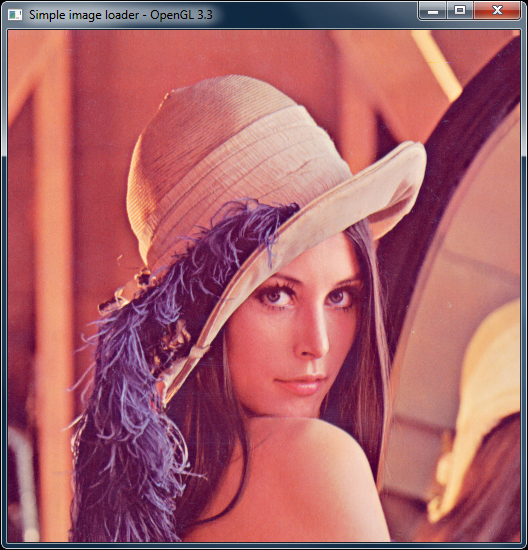Using image loading libraries like `SOIL` and a fragment shader, we can make a simple image viewer with basic GLSL functionality. More elaborate effects may be achieved by using techniques detailed in the later recipes of this book.